# Solution assignment 09 Fractional functions and graphs

### Assignment 9

Investigate the function: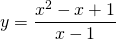and sketch its graph.

### Solution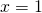is a vertical asymptote (forthe function becomes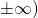, but this is not the case as we will see later. When we want to find the horizontal asymptote we encounter some problems. For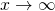of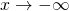the function approaches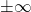. In order to get more information of the behavior of the function forof, we rewrite the function as follows: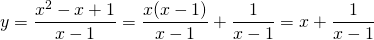We see that the function approaches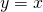whenor. This means that the function approaches the line:.
We also notice that the intersection point with the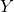-axis is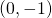and we find the intersection point with the-axis by solving the equation which we get by taking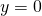. So we have to solve the following equation: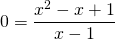If a fraction equals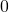then the numerator equalsand thus we have to solve:This is a quadratic equation. The discriminant: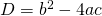is less than. The equation has no solutions and thus the graph has no intersection points with the-axis.
Using calculus we can also calculate by differentiation for which values ofthe graph has a maximum or minimum, but we do not do this here. If you know enough of differentiation, please do this yourself.
We have enough information to sketch the graph. In the figure both the graph and the lineare depicted.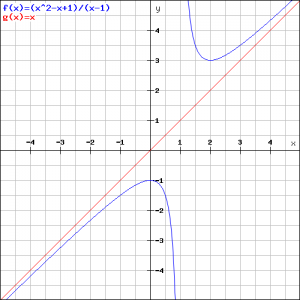0